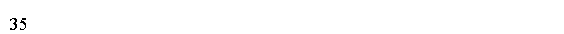# Java 流操作

2018-03-12 16:39 更新

## 外部迭代

```import java.util.Arrays;
import java.util.List;

public class Main {
public static void main(String[] args) {
List<Integer> numbers = Arrays.asList(1, 2, 3, 4, 5);
int sum = 0;
for (int n : numbers) {
if (n % 2 == 1) {
int square = n * n;
sum = sum + square;
}
}
System.out.println(sum);
}
}
```## 内部迭代

```import java.util.Arrays;
import java.util.List;

public class Main {
public static void main(String[] args) {
List<Integer> numbers = Arrays.asList(1, 2, 3, 4, 5);
int sum = numbers.stream()
.filter(n -> n % 2  == 1)
.map(n  -> n * n)
.reduce(0, Integer::sum);

System.out.println(sum);
}
}
```## 顺序

```import java.util.Arrays;
import java.util.List;

public class Main {
public static void main(String[] args) {
List<Integer> numbers = Arrays.asList(1, 2, 3, 4, 5);
int sum = numbers.parallelStream()
.filter(n -> n % 2  == 1)
.map(n  -> n * n)
.reduce(0, Integer::sum);

System.out.println(sum);
}
}
```## 命令式与功能式

Streams API通过使用lambda表达式支持函数式编程。

## 中间业务终端业务

• 中间操作
• 终端操作

Streams链接操作以创建流管道。

```import java.util.Arrays;
import java.util.List;

public class Main {
public static void main(String[] args) {
List<Integer> numbers = Arrays.asList(1, 2, 3, 4, 5);
int sum = numbers.parallelStream()
.filter(n -> n % 2  == 1)
.map(n  -> n * n)
.reduce(0, Integer::sum);

System.out.println(sum);
}
}
```App下载